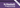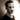Back to basicsDon't worry: we're getting there!

Welcome to the second part of reading Haskell Programming from First Principles. This time around we finally see some actual Haskell code. Sort of. It's mostly just a tour of Haskell's basic syntax, along with a brief introduction to the REPL and how that works. As such, let's do a quick recap of the most essential parts.

`There are quite a few references to /the REPL/ in this article. /REPL/ is short for /read eval print loop/ and is a command line interpreter for a programming language. In our case, GHC's REPL is invoked by either the ~ghci~ or the ~stack ghci~ command, depending on whether you're using GHC directly or through Stack.`

## General notes

First off, Haskell is a whitespace-sensitive language. Much like in Python, the indentation of your code matters. A lot. However, unlike in Python, indentation doesn't come in multiples of four. Or two. Or any number, really. Instead, it's dependent on the code (and as such, tabs are out). As the book states:

The basic rule is that code that is part of an expression should be indented under the beginning of that expression, even when the beginning of the expression is not at the leftmost margin. Furthermore, parts of the expression that are grouped should be indented to the same level.

To make that clearer, let's have an example:

``````calc x =
let y = x ^ 2
z = x - 1
in x + y + z``````

Notice how all the variable assignments line up and how the `in` block (or line in this case) is indented one space more than the `let` block. Like many languages, Haskell has multiple valid ways to structure your code, so just find a style you like and stick to it. The key thing is: don't freak out if the indentation isn't what you expect at first.

Point two: capitalization matters. Haskell is quite strict about this and won't compile unless you follow the rules. Functions and variables start with lowercase letters (and conventionally use camelCase for longer identifiers), while types and type constructors (e.g. `Bool` and its variants `True` and `False`, `Int`, etc.) are captitalized (and use what's sometimes known as PascalCase).

Point three: Haskell is 'lazy', or 'lazily evaluated' or 'non-strict'. What this means is that Haskell won't evaluate anything until it really needs to. This is why we can work with infinite lists with no problems---unless you try and consume the whole thing, of course.

Remember when we talked about beta normal form and beta reduction in the last chapter? Haskell doesn't evaluate everything to normal form by default. Instead, it evaluates to what is known as weak head normal form. Because of referential transparency, it knows that it can compute everything on demand, so until a value is required from an expression, the expression will be left unevaluated. Imagine it's a pointer to an expression instead of a value, which can be lazily evaluated when it's first used.

Finally, on comment syntax: Haskell single-line comments start with a double dash (a literal `--`, not the Nintendo kart racing kind), while multiline comments are put between `{-` and `-}`.

## Functions

Now, let's have a closer look at function definitions. A function definition is made up of the name of the function, the parameters (separated by whitespace), an equals sign, and the expression that is the body. Example:

``add x y = x + y``

This function takes two arguments---~x~ and `y`---and returns the sum. Notice that we don't say anything about the types here. The compiler is smart enough to figure that out. If we use the REPL and the `:info` (or `:i`) command to describe this function, it tells us that the type of the expression is: `Num a => a -> a -> a`. It's automatically generic for all numeric types (well, all instances of the typeclass `Num`, but we're getting ahead of ourselves). Neat!

We won't be looking any deeper into type annotations and function signatures for the time being. They're not covered in this chapter, but do show up later; so if all the arrows in the the type signature above confuse you: don't worry.

It's also worth mentioning that, much like lambda calculus, Haskell uses curried functions. If you forgot what that means, the authors describe it like this: "In Haskell, when it seems we are passing multiple arguments to a function, we are actually applying a series of nested functions, each to one argument." Because we return a new function for each argument we apply, we can assign the result of a partially applied function to a variable and save it for later, just like we did with the lambda expressions last time.

### Operators and infix functions

In addition to regular functions, Haskell also has operators. These are a mix of what you'd expect from any programming language (`+`, `-`, `*`, `/`, etc.) and more exotic ones (`>>=`, `<\$>`). In fact, Haskell lets you define your own operators too. This might sound unconventional to some, but when you realize that a binary operator is really just a binary function placed in between its arguments, it makes a lot of sense.

See, binary operators are what we call infix, which means that they go in between their arguments. But operators aren't the only things that can be infix: Any binary function in Haskell can be used as an infix function if you wrap it in backticks (e.g. `div 2 2` becomes `2 `div` 2`).

Using partially applied infix operators is called sectioning. It's briefly mentioned in the book and there's also a very easily understood article on the Haskell wiki on it, so let's use an extract from the latter to clarify:

Essentially, you only give one of the arguments to the infix operator, and it represents a function which intuitively takes an argument and puts it on the "missing" side of the infix operator. - ~(2^)~ (left section) is equivalent to ~(^) 2~, or more verbosely ~\x -> 2 ^ x~ - ~(^2)~ (right section) is equivalent to ~flip (^) 2~, or more verbosely ~\x -> x ^ 2~

When working with operators, it's important to keep in mind the precedence rules. Most of us probably know that multiplication has higher precedence than addition, for instance---which is why \$1+3*5\$ is \$16\$ and not \$20\$---but it's not always immediately obvious for all operators. In Haskell, precedence is defined as a number between 0 and 9, where higher numbers denote higher precedence. If you ever wonder about a specific operator, you can use the REPL's `:info` command to get information about precedence for any operator (e.g. `:i (*)`).

Most of the operators introduced in this chapter are pretty self-explanatory---don't worry, the weird ones I referenced before aren't mentioned---but there is one that is singled out into its own little section: `\$`. This operator is a little special in that it has a precedence of 0 and that it is defined as `f \$ a = f a`.

What?

Indeed, it doesn't actually do anything, but it's all about the precedence. This allows us to use this operator to write an expression (`a`) after it that will get fed into the expression before it (`f`).

Err ... okay? So what's the point?

Oh, it helps us avoid parentheses and a lot of nesting of functions. It's very useful for composition. A contrived example would be:

``````-- is the result of the calculation even?

-- without the \$ symbol
even (2 + 2)

-- with the \$ symbol
even \$ 2 + 2``````

It probably still seems pretty pointless, but you'll grow to appreciate it as we progress further. Trust me.

### Assigning variables in expressions

As we saw in the first section, you can assign variables with a `let` expression, but there is another way too: the `where` declaration.

`let` introduces an expression, and can thus be used wherever you can use expressions, while `where` is a syntactic construct, and is only valid in certain parts of the code. If you're in a function, though, both will serve you just fine. These two functions evaluate to the same result:

``````letFunction x =
let y = x + 1
in y + x

whereFunction x = y + x
where
y = x + 1``````

Apart from mentioning that a `let` expression is just that, an expression, and that `where` is a declaration, the book doesn't go into any more details of when to prefer one over the other, so neither will we. However, if you're interested, I encourage you to check out the Let vs. Where article on the Haskell wiki.

## Definitions

Definition time! This is a selection of the definitions from the end of the chapter, leaving out the definitions not related to what we have touched on.

Expression
Anything that conforms to the Haskell syntax and that can be reduced to a result. In theory, even constants that can not be reduced (such as the number \$1\$) are expressions, but these are generally referred to as values in common parlance.
Value
A value is an expression that can not be reduced further.
Function
A mathematical object that maps a set of inputs (the domain) to a set of outputs (the codomain). A transformer of values. It might be interesting to note that the book also mentions that functions can be described as a list of ordered pairs of their inputs and corresponding outputs: Example: A function `(^2)` (a simple square function) defined for natural numbers would start with the entries `(0, 0)`, `(1, 1)`, `(2, 4)`, `(3, 9)`
Infix notation
A style of notation where the operator is placed between the operands.
Operators
In Haskell: Functions that are infix by default. Must use symbols only.

## Conclusion and next time

Not bad! We've seen some actual code this time and played around a bit at the REPL. We learned a bit about the basic syntax and about how operators and infix notation work, about sectioning and the `\$` operator.

Next time, we'll be looking at the `String` type and how it is implemented in Haskell.

See you then!Thomas Heartman is a developer, writer, speaker, and one of those odd people who enjoy lifting heavy things and putting them back down again. Preferably with others. Doing his best to gain and share as much knowledge as possible.